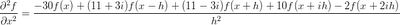## Friday, December 5, 2008

### Asymmetric Complex Step

To solve Laplace's equation with a complex step finite difference we needed to develop asymmetric difference relations to solve on the +/- imaginary planes.

On the plane in the negative imaginary direction we difference centrally in the real direction and forward two steps in the imaginary direction:And on the plane in the positive imaginary direction we difference centrally in the real direction and backward two steps in the imaginary direction:We still want the real part of the answer as we did before, but now we have imaginary coefficients for our function values in the real direction. While we have a compact stencil on the real plane (only nearest neighbors are used), we lose that property on either imaginary plane of the solution. This means our system matrix bandwidth is larger than it would be if we had a purely compact stencil for all parts of the solution.

It's fairly easy to calculate arbitrary derivative approximation formulas using Maxima.

/* calculate the coefficients for a finite difference approximation of
a derivative, can include complex steps */

steps : matrix([1,2,%i,2*%i]); /* multiple of the step size h */

/* sum df d2f d3f d4f */
derivs : matrix(, , , , ); /* the rhs for our system */

n : matrix_size( steps );

A : zeromatrix(n+1,n+1);

for j : 1 thru n+1 do
( A[1,j] : 1,
for i : 2 thru n+1 do
(
if j = 1 then A[i,j] : 0 else A[i,j] : steps[1,j-1]^(i-1)/(i-1)!
)
);

invA : invert(A);

coeffs : expand( invA.derivs );
expand( 20*coeffs );

Where the vector steps tells which points will be included in the stencil, and the vector derivs tells which derivative we want to approximate. This example is calculating a real and complex forward difference approximation for the first derivative.

You can download this Maxima batch file here. This example can be run from the command line:

\$ maxima -b fd.mac

or using the batch command from an interactive Maxima session:

(%i1) batch("fd.mac")